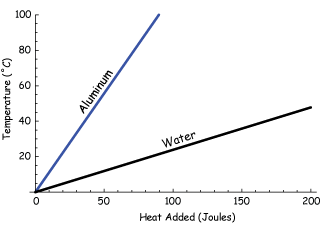# Investigate Specific Heat Capacity Required Practical

## Experimental Set Up

Investigating the specific heat capacity of a substance is a required practical!

The experimental set-up looks like this:We have:

1. Copper block wrapped in insulation, with two holes for a thermometer and heater
2. Thermometer
3. Pipette to put oil in the thermometer hole
4. 30 W heater
5. 12 V power supply
6. Ammeter
7. Insulation to wrap around the blocks
8. Stop watch or stop clock

## Method

1. Measure and record the mass of the copper block in kg.
2. Place a heater in the larger hole in the block.
3. Use the pipette to put a small amount of oil in the hole.
4. Put the thermometer in this hole.
5. Switch the power pack to 12V. Switch it on.
6. Measure the temperature and switch on the stop clock.
7. Record the temperature every minute for 10 minutes.
8. Calculate the power of the heater in Watts. To do this, multiply the ammeter reading by the voltmeter reading.
9. Calculate the work done by the heater in Joules. To do this, multiply the time in seconds by the power of the heater.

## Graph

The graph would have the energy supplied on the x axis and the temperature on the y axis.Specific heat capacity could be calculated by finding the gradient of the line. This can be done by the finding the change in y and dividing it by the change in x.

How do you calculate specific heat capacity from the graph?